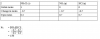#### Metanoia

When solid ammonium chloride dissociates at a certain temperature in a 0.500dm3 container, ammonia and hydrogen chloride are formed. NH4Cl(s) NH3(g) + HCl(g) The initial amount of ammonium chloride was 1.00mol, and when the system had reached equilibrium there was 0.300mol of ammonium chloride. What is the numerical value of Kc for this reaction under these conditions?
A 0.490 B 1.63 C 1.96 D 3.27
can u show me how to sove it??
Note that ammonium chloride does not appear in the Kc expression as it is a SOLID.•Asim Shah

#### Ahsan Ausaf Ali

Been a long time since I left tho forum but I see A Levels is still all the same, nostalgia !

•Asim Shah

#### A*****

Been a long time since I left tho forum but I see A Levels is still all the same, nostalgia !
Hahahaha so what r u doing nowadays?

#### Ahsan Ausaf Ali

Hahahaha so what r u doing nowadays?
done with engineering obviously, A Levels was great though, and this forum used to be a life saver, i remember just 30 miinutes after each paper, we all used to be here discussing Lol.... what a feeling !

•Asim Shah and A*****

#### feliciablack2000

The equation constant for the following reaction is 1.39 ×10^-2
2HI ⇌ H^2 + I^2
What will be the equilibrium constant for the following reaction?
H^2+ I^2 ⇌ 2HI
How do i solve this??

#### A*****

The equation constant for the following reaction is 1.39 ×10^-2
2HI ⇌ H^2 + I^2
What will be the equilibrium constant for the following reaction?
H^2+ I^2 ⇌ 2HI
How do i solve this??
What do u mean by equation constant?

•Hamnah Zahoor

#### Hamnah Zahoor

What do u mean by equation constant?
I guess equilibrium constant is sometime called equation constant in this case Kc.

•A*****

#### A*****

The equation constant for the following reaction is 1.39 ×10^-2
2HI ⇌ H^2 + I^2
What will be the equilibrium constant for the following reaction?
H^2+ I^2 ⇌ 2HI
How do i solve this??
Won't it be the reciprocal of 1.39 x 10^-2 i.e. 71.9?

#### Hamnah Zahoor

The equation constant for the following reaction is 1.39 ×10^-2
2HI ⇌ H^2 + I^2
What will be the equilibrium constant for the following reaction?
H^2+ I^2 ⇌ 2HI
How do i solve this??

#### Hamnah Zahoor

Won't it be the reciprocal of 1.39 x 10^-2 i.e. 71.9?
I don't think it will be reciprocal ....when writing initial moles and the final moles of the first equation
x2
(1-2x)2
=1.39*10-2

for the next reaction
(2x)2
(1-x)(1-x)
=?
and i am getting Kc=4.51*10-2

#### A*****

I don't think it will be reciprocal ....when writing initial moles and the final moles of the first equation
x2
(1-2x)2
=1.39*10-2

for the next reaction
(2x)2
(1-x)(1-x)
=?
and i am getting Kc=4.51*10-2
When u take the moles for any product to be x in the first reaction, then y don't u take it as x in the next reaction, where it becomes the moles of the reactant?

•Hamnah Zahoor

#### Hamnah Zahoor

When u take the moles for any product to be x in the first reaction, then y don't u take it as x in the next reaction, where it becomes the moles of the reactant?
Taking it as X in the reactants of the reaction will show that the number of moles at equilibrium and before equilibrium are same. In order to obtain moles at equilibrium we subtract the initial moles, which is considered to be '1' from the unknown decrease stated as 'X' after equilibrium is reached (1-x). However product will be taken as '2X' after equilibrium is reached.

Last edited:

#### Metanoia

Won't it be the reciprocal of 1.39 x 10^-2 i.e. 71.9?
Yes, that is correct. The Kc of the forward reaction is the reciprocal of the backward reaction.

•A***** and Hamnah Zahoor

#### Hamnah Zahoor

Yes, that is correct. The Kc of the forward reaction is the reciprocal of the backward reaction.
Thank You Sir, for clarifying it.

Last edited:
•Metanoia

#### feliciablack2000

I am sorry i didnt share any details of the question. The question is actually from Sir Rizwan's past paper booklet and it is not in any past paper. The answer is 71.94.

#### joseph charlie

Please hhelp with this question for may june 2006

Use of the Data Booklet is relevant to this question.

What mass of solid residue can be obtained from the thermal decomposition of 4.10

g of
anhydrous calcium nitrate?

A 0.70

g B 1.00g

C 1.40g

D 2.25g

#### A*****

Please hhelp with this question for may june 2006

Use of the Data Booklet is relevant to this question.

What mass of solid residue can be obtained from the thermal decomposition of 4.10

g of
anhydrous calcium nitrate?

A 0.70

g B 1.00g

C 1.40g

D 2.25g
Moles of calcium nitrate= 4.10/164.1 = 0.02498
Moles of calcium oxide= 0.02498
Mass of calcium oxide= 0.02498 x 56.1=1.40g

#### joseph charlie

Thanks for the help i appreciate it , but i kinda figured it out on my own after reading nd understanding the question.

•A*****